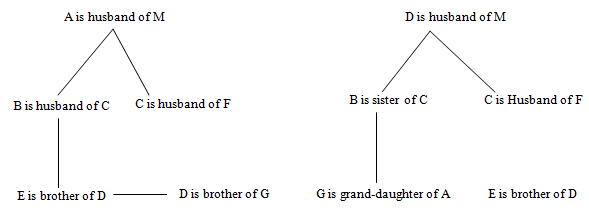# Wipro Reasoning Questions

Logical Reasoning is an essential component of Wipro recruitment test. It includes questions from typical topics like Blood Relations, Arrangements, word sequence, data sufficiency, Syllogism, series, coding-decoding, etc. This part is devised to check the deductive, inductive, and abductive reasoning skills of the candidates. Reasoning section has both sets and standalone questions. Here are some sample reasoning questions that appear in placement papers of top companies like Wipro:
###### Wipro Reasoning Questions
DIRECTION for questions 1 to 3: Study the given information carefully and answer the given questions:
A is the mother of B. B is the sister of C. D is the son of C. E is the brother of D. F is the mother of E. G is the granddaughter of A. H has only two children – B and C.
1. How is F related to H ?
1. Son-in-law
2. Daughter in-law
3. Granddaughter
4. Cannot be determinedFrom above figure it is clear that H is mother of C & F is wife of C so daughter in law, hence 2nd option is the correct answer.
2. How is C related to E?
1. Father
2. Son
3. Cousin brother
4. Cannot be determinedFrom figure it is clear that C is father of E. So, the correct answer is option A
3. Who is the mother of G?
1. G
2. B
3. F
4. Either B or FClearly, Mother of G will be either B or F
Hence, the answer is option D.
DIRECTION for questions 4 to 6: The following questions are based on the five three letter words given below:
FAN HOP GET CUB MID
(NOTE : The words formed after performing the given operations may or may not be meaningful English words.)
1. If the positions of the first and second letters of all the words are interchanged, how many words will form meaningful English words ?
1. None
2. Three
3. One
4. Two
F Words will be AFN,OHP,EGT,UCB,IMD, none of these is a meaningful word. So, the correct answer is option A
2. If all the letters in each of the words are arranged alphabetically (within the word), how many words will remain unchanged?
1. Two
2. Three
3. One
4. None
FAN - AFN
HOP - HOP
GET - EGT
CUB - BCU
MID - DIM
HOP remains same
Therefore, the correct answer is option C.
3. If the first letter of all the words is changed to the next letter of the English alphabetical series, how many words will have more than one vowel ? (Same or different vowel)
1. None
2. Three
3. Two
4. More than three
5. One
From the given information, we have
Words will be GAN IOP HET DUB NID, out of these five words, only IOP has two vowels.
Hence, the correct answer is option E
4. Which of the following will come next in the following series?
a z a b y a b c x a b c d w a b c d
1. f
2. u
3. a
4. y
5. e
a, z, ab, y, abc, x, abcd, w…next will be abcde.
So, the next alphabet will be 'e'
Hence, the correct answer is option E.

5. In a certain code language, TONIC is coded as 'CINOT' and 'SCALE' is coded as 'ACELS'. In the same code language, 'PLANK' will be coded as
1. KNALP
2. AKNLP
3. AKLNP
4. AKPNL
5. Cannot be determined
Letters are alphabetically arranged.
Therefore, 'PLANK' will be coded as 'AKLNP'
So, the correct answer is option C.
6. Which of the following will come in the place of the question mark (?) in the following series based on the English alphabetical order ?
YS RM LH ? CA
1. DG
2. FD
3. GD
4. DF
Here we have
Y(25) to S(19)…difference -6
R(18) to M (13)..difference -5
L(12) to H(8)…..difference -4
Similarly. G(7) to D(4) …….difference -3
C(3) to A(1) ………difference -2
So, the correct answer is option C.
7. How many such pairs of letters are there in the word NEUTRAL, each of which has as many letters between them in the word (in both forward and backward directions) as they have between them in the English alphabetical series ?
1. Two
2. Three
3. None
4. One